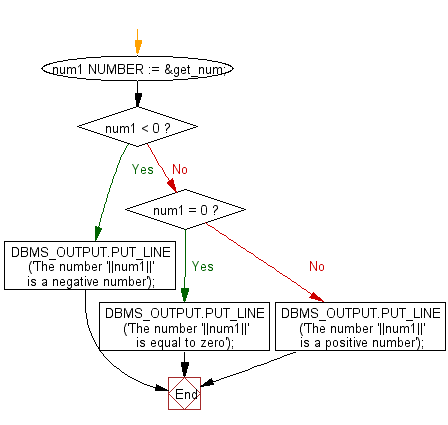﻿ PL/SQL Control Statement: Check whether a given number is positive, negative or zero - w3resource# PL/SQL Control Statement Exercises: Check whether a given number is positive, negative or zero

## PL/SQL Control Statement: Exercise-12 with Solution

Write a PL/SQL program to check whether a given number is positive, negative or zero.

Sample Solution:

PL/SQL Code:

``````DECLARE
num1 NUMBER := &get_num;
BEGIN
IF num1 < 0 THEN
DBMS_OUTPUT.PUT_LINE ('The number '||num1||' is a negative number');
ELSIF num1 = 0 THEN
DBMS_OUTPUT.PUT_LINE ('The number '||num1||' is equal to zero');
ELSE
DBMS_OUTPUT.PUT_LINE ('The number '||num1||' is a positive number');
END IF;
END;
/
```
```

Sample Output:

```SQL> /
Enter value for get_num: 45
old   2: num1 NUMBER := &get_num;
new   2: num1 NUMBER := 45;
The number 45 is a positive number

PL/SQL procedure successfully completed
```

Sample Output:

```SQL> /
Enter value for get_num: 0
old   2: num1 NUMBER := &get_num;
new   2: num1 NUMBER := 0;
The number 0 is equal to zero

PL/SQL procedure successfully completed.
```

Sample Output:

```SQL> /
Enter value for get_num: -15
old   2: num1 NUMBER := &get_num;
new   2: num1 NUMBER := -15;
The number -15 is a negative number

PL/SQL procedure successfully completed.
```

Flowchart:Improve this sample solution and post your code through Disqus

What is the difficulty level of this exercise?

﻿